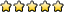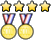## Astronomy C

Unome
ModeratorPosts: 4209
Joined: January 26th, 2014, 12:48 pm
State: GA
Location: somewhere in the sciolyverse

### Re: Astronomy C

y_utsumi wrote:How can this be solved? I know it has to do with Kepler's laws, but I keep getting a very large answer..

Suppose that the mass of Sirius B is 0.98 solar masses, and the semi-major axis between Sirius A is 7.5 arcsec observed from Earth, and that we are viewing the system face-on. Calculate the period for the Sirius system to complete one orbit in Earth years.
(The previous questions gave the following information: mass of Sirius A = 2.18 solar masses, Sirius parallax (observed from Earth) = 0.38 arcsec, apparent magnitude of Sirius A = -1.47.)

The answer is 48.4 +/- 1.0 years.
Parallax to distance: 1 / 0.38 = 2.6315 pc

Set up a triangle, and the semi major axis = 2.6315 * tan ( 7.5 arcsec ) = 9.568 * 10^-5 parsecs = 19.73625 AU

Since p^2 = a^3 when period is in years and length of the semi-major axis is in then:
p = sqrt ( a^3 )
p = sqrt ( 19.73625^3 )
period = 87.68 years

(yay I can do these now!)

Magikarpmaster629
Exalted MemberPosts: 578
Joined: October 7th, 2014, 3:03 pm
State: MA
Location: No idea, but I can tell you exactly how fast I'm going

### Re: Astronomy C

Unome wrote:
y_utsumi wrote:How can this be solved? I know it has to do with Kepler's laws, but I keep getting a very large answer..

Suppose that the mass of Sirius B is 0.98 solar masses, and the semi-major axis between Sirius A is 7.5 arcsec observed from Earth, and that we are viewing the system face-on. Calculate the period for the Sirius system to complete one orbit in Earth years.
(The previous questions gave the following information: mass of Sirius A = 2.18 solar masses, Sirius parallax (observed from Earth) = 0.38 arcsec, apparent magnitude of Sirius A = -1.47.)

The answer is 48.4 +/- 1.0 years.
Parallax to distance: 1 / 0.38 = 2.6315 pc

Set up a triangle, and the semi major axis = 2.6315 * tan ( 7.5 arcsec ) = 9.568 * 10^-5 parsecs = 19.73625 AU

Since p^2 = a^3 when period is in years and length of the semi-major axis is in then:
p = sqrt ( a^3 )
p = sqrt ( 19.73625^3 )
period = 87.68 years

(yay I can do these now!)
Well, not quite. You got Kepler's law right for the specific case of the solar system, but remember the mass of the system is important.
This is the general form of Kepler's Third:

Where P is period, G is Newton's Gravitational Constant, M is the mass of the system, and a is period (all in SI/metric units).
Or, the form I like it in:

Where P is period in years, M is system mass in solar masses, and a is the semi-major axis in AU.
You didn't take mass into account, so let's do that here:
(yay LaTeX!)

y_utsumi
MemberPosts: 6
Joined: August 2nd, 2016, 8:14 pm
Division: C
State: FL

### Re: Astronomy C

Unome wrote:
y_utsumi wrote:How can this be solved? I know it has to do with Kepler's laws, but I keep getting a very large answer..

Suppose that the mass of Sirius B is 0.98 solar masses, and the semi-major axis between Sirius A is 7.5 arcsec observed from Earth, and that we are viewing the system face-on. Calculate the period for the Sirius system to complete one orbit in Earth years.
(The previous questions gave the following information: mass of Sirius A = 2.18 solar masses, Sirius parallax (observed from Earth) = 0.38 arcsec, apparent magnitude of Sirius A = -1.47.)

The answer is 48.4 +/- 1.0 years.
Parallax to distance: 1 / 0.38 = 2.6315 pc

Set up a triangle, and the semi major axis = 2.6315 * tan ( 7.5 arcsec ) = 9.568 * 10^-5 parsecs = 19.73625 AU

Since p^2 = a^3 when period is in years and length of the semi-major axis is in then:
p = sqrt ( a^3 )
p = sqrt ( 19.73625^3 )
period = 87.68 years

(yay I can do these now!)
Thanks! Your logic worked, except Kepler's 3rd law discusses the proportional relationship between p^2 and a^3. We ended up setting total mass equal to (a^3)/(p^2) and got 49.3 years, which is in their range of accepted answers.

jonboyage
MemberPosts: 104
Joined: December 13th, 2016, 8:32 am
State: PA

### Re: Astronomy C

How do you find the minimum distance between a white dwarf and a red giant so that mass transfer can occur?

ModeratorPosts: 485
Joined: December 6th, 2013, 1:56 pm
State: TX
Location: Austin, Texas

### Re: Astronomy C

jonboyage wrote:How do you find the minimum distance between a white dwarf and a red giant so that mass transfer can occur?
It's twofold, at least from what I understand of mass transfer in binary systems (although admittedly, I'm not that good at it).

Mass transfer occurs when either of these two things happen:

1. the star expands to fill the Roche lobe physically, due to stellar evolution (think of a star becoming huge after it leaves the main sequence and becomes a red giant)
2. the orbit becomes smaller so that one star is inside of the other star's Roche lobe, due to angular momentum loss (e.g magnetic braking)

So essentially, it would be the radius of the Roche lobe, from what I know. It's not very exact, and the shape varies depending on the stars, of course, but you can find approximations on Wikipedia.

Magikarpmaster629
Exalted MemberPosts: 578
Joined: October 7th, 2014, 3:03 pm
State: MA
Location: No idea, but I can tell you exactly how fast I'm going

### Re: Astronomy C

jonboyage wrote:How do you find the minimum distance between a white dwarf and a red giant so that mass transfer can occur?
It's twofold, at least from what I understand of mass transfer in binary systems (although admittedly, I'm not that good at it).

Mass transfer occurs when either of these two things happen:

1. the star expands to fill the Roche lobe physically, due to stellar evolution (think of a star becoming huge after it leaves the main sequence and becomes a red giant)
2. the orbit becomes smaller so that one star is inside of the other star's Roche lobe, due to angular momentum loss (e.g magnetic braking)

So essentially, it would be the radius of the Roche lobe, from what I know. It's not very exact, and the shape varies depending on the stars, of course, but you can find approximations on Wikipedia.
I think the radius of the roche lobe is just the first lagrange point; that's the point at which all the mass in the mass transfer is focused through before being pulled into an accretion disk.

ModeratorPosts: 485
Joined: December 6th, 2013, 1:56 pm
State: TX
Location: Austin, Texas

### Re: Astronomy C

Magikarpmaster629 wrote:
jonboyage wrote:How do you find the minimum distance between a white dwarf and a red giant so that mass transfer can occur?
It's twofold, at least from what I understand of mass transfer in binary systems (although admittedly, I'm not that good at it).

Mass transfer occurs when either of these two things happen:

1. the star expands to fill the Roche lobe physically, due to stellar evolution (think of a star becoming huge after it leaves the main sequence and becomes a red giant)
2. the orbit becomes smaller so that one star is inside of the other star's Roche lobe, due to angular momentum loss (e.g magnetic braking)

So essentially, it would be the radius of the Roche lobe, from what I know. It's not very exact, and the shape varies depending on the stars, of course, but you can find approximations on Wikipedia.
I think the radius of the roche lobe is just the first lagrange point; that's the point at which all the mass in the mass transfer is focused through before being pulled into an accretion disk.
I didn't know that; thanks!It makes sense though, considering the definition of a Lagrange point and Roche lobes and all that

For those wondering:
wikipedia wrote:[The Roche lobe] is an approximately tear-drop-shaped region bounded by a critical gravitational equipotential, with the apex of the tear drop pointing towards the other star (the apex is at the L1 Lagrangian point of the system).

jonboyage
MemberPosts: 104
Joined: December 13th, 2016, 8:32 am
State: PA

### Re: Astronomy C

Thanks for the responses guys!

I was actually looking for something a little bit different. I was wondering how you would actually calculate the location of the Lagrange point if you just know the masses of the stars and their sizes.

Thanks again!

Unome
ModeratorPosts: 4209
Joined: January 26th, 2014, 12:48 pm
State: GA
Location: somewhere in the sciolyverse

### Re: Astronomy C

jonboyage wrote:Thanks for the responses guys!

I was actually looking for something a little bit different. I was wondering how you would actually calculate the location of the Lagrange point if you just know the masses of the stars and their sizes.

Thanks again!
There are some approximations on the Wikipedia page (I just skimmed the page briefly though). I'm fairly sure that there's no general solution to finding the first three Lagrangian points.

ModeratorPosts: 485
Joined: December 6th, 2013, 1:56 pm
State: TX
Location: Austin, Texas

### Re: Astronomy C

jonboyage wrote:Thanks for the responses guys!

I was actually looking for something a little bit different. I was wondering how you would actually calculate the location of the Lagrange point if you just know the masses of the stars and their sizes.

Thanks again!
As Unome said, you can find approximations and such on wikipedia:### Who is online

Users browsing this forum: No registered users and 1 guest